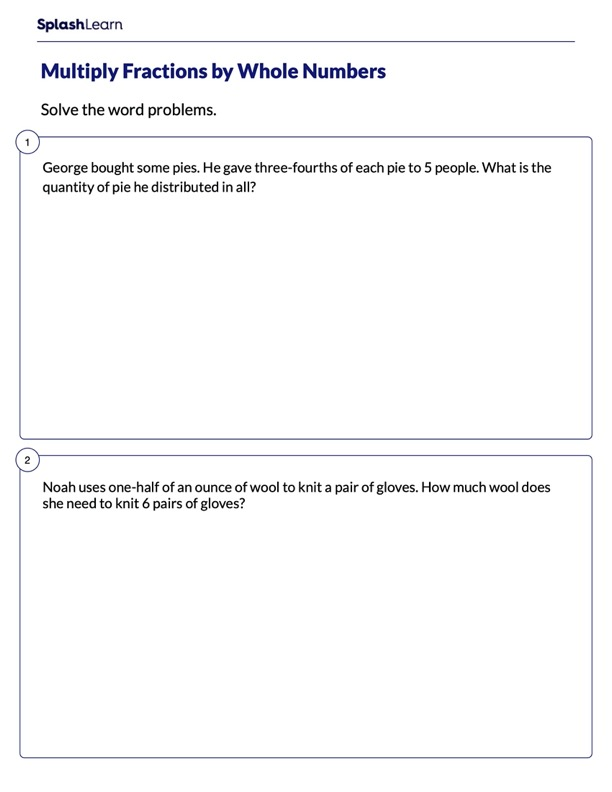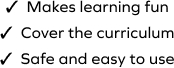# Word Problems Multiplying Fraction by Whole Number

SplashLearn - The complete PreK - Grade 5 Math & ELA Learning Program Built for Your Child
Home > Word Problems Multiplying Fraction by Whole NumberHelp your child learn the multiplication of fractions in an efficient manner with this challenging worksheet. Students will strengthen their problem-solving ability by working with word problems on multiplication of fractions in this worksheet. They will on a set of multiply by scenarios and find the unknown quantity. This worksheet will help your students learn multiplication of fractions by whole numbers in an efficient manner.